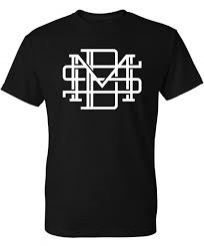# Different 6709

Milan found out that he could wear pants and a T-shirt in a total of 28 different ways. How many T-shirts and pants can he have? List all options.

t1 =  1
n1 =  28
t2 =  2
n2 =  14
t3 =  4
n3 =  7
t4 =  7
n4 =  4
t5 =  14
n5 =  2
t6 =  28
n6 =  1

### Step-by-step explanation:Did you find an error or inaccuracy? Feel free to write us. Thank you!

Tips for related online calculators